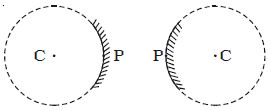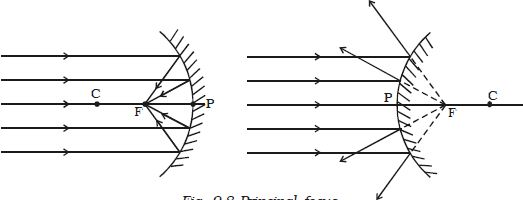Physics

# How Reflection occur at Curved Surfaces

Reflection at Curved Surfaces:

In optics here concerned with curved mirrors which are the part of a hollow sphere (figure). One surface of the mirror is silvered. Reflection takes place at the other surface. If the reflection takes place at the concave surface, (which is towards the centre of the sphere) it is called concave mirror.Fig: concave and convex mirror

If the reflection takes place at the convex surface, (which is away from the centre of the sphere) it is called convex mirror. The laws of reflection at a plane mirror are equally true for spherical mirrors also.

The centre of the sphere, of which the mirror is a part, is called the centre of curvature (C).

The geometrical centre of the mirror is called its pole (P).

The line joining the pole of the mirror and its centre of curvature is called the principal axis.

The distance between the pole and the centre of curvature of the spherical mirror is called the radius of curvature of the mirror and is also equal to the radius of the sphere of which the mirror forms a part.When a parallel beam of light is incident on a spherical mirror, the point where the reflected rays converge (concave mirror) or appear to diverge from the point (convex mirror) on the principal axis is called the principal focus (F) of the mirror. The distance between the pole and the principal focus is called the focal length (f) of the mirror (Figure).